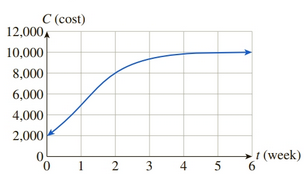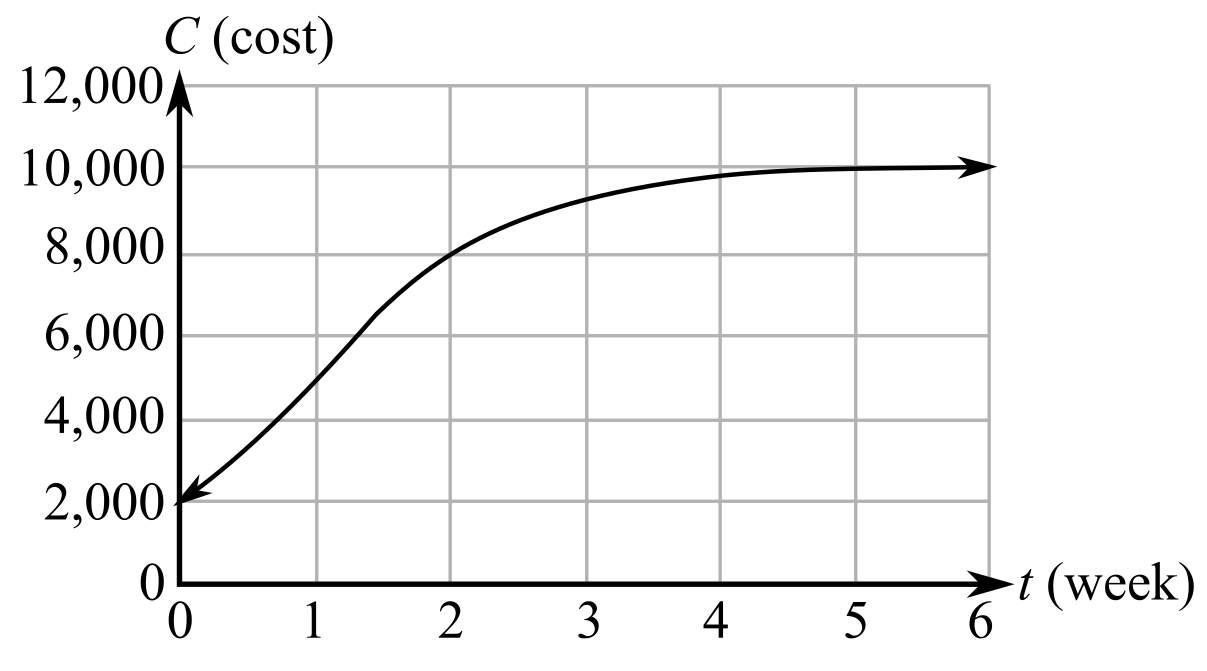Chapter 10, Problem 56REFinite Mathematics and Applied Cal...

7th Edition
Stefan Waner + 1 other
ISBN: 9781337274203

Solutions

Chapter
SectionFinite Mathematics and Applied Cal...

7th Edition
Stefan Waner + 1 other
ISBN: 9781337274203
Textbook Problem

Advertising Costs The following graph (see Exercise 52) shows the weekly cost of OHaganBooks.com’s online ad campaign for the 6-week period since the start of July (t is time in weeks)Use the graph to answer the following questions:a. What was the average rate of change of cost over the entire 6-week period?b. What was the average rate of change of cost over the period [ 2 , 6 ] ?c. Which of the following is correct? Over the period [ 2 , 6 ] (A) the rate of change of cost increased and the cost increased.(B) the rate of change of cost decreased and the cost increased.(C) the rate of change of cost increased and the cost decreased.(D) the rate of change of cost decreased and the cost decreased.

(a)

To determine

To calculate: The average rate of change of the cost of online ad campaign of OHaganBooks.com in time interval of 6 week with the help of graph provided. The graph is:Explanation

Given information:

The graph of the cost of online ad campaign of OHaganBooks.com in time interval of 6 week is provided as:

Formula used:

The average rate of change of function f(x) is:

average rate of change=ΔfΔt

Calculation:

Consider the period of 6 weeks that is [0,6]

From the graph, the value of C(0)=2000 and C(6)=10000

(b)

To determine

To calculate: The average rate of change of the cost of online ad campaign of OHaganBooks.com in time interval of [2,6] with the help of graph provided. The graph is:(c)

To determine

The correct options for the time interval [2,6] with the help of graph provided that represents the cost of online ad campaign of OHaganBooks.com in time interval of 6 week out of the following options.

The options are:

The rate of change of the cost increased and the cost increased.

The rate of change of the cost decreased and the cost increased.

The rate of change of the cost increased and the cost decreased.

The rate of change of the cost decreased and the cost decreased.

Still sussing out bartleby?

Check out a sample textbook solution.

See a sample solution

The Solution to Your Study Problems

Bartleby provides explanations to thousands of textbook problems written by our experts, many with advanced degrees!

Get Started